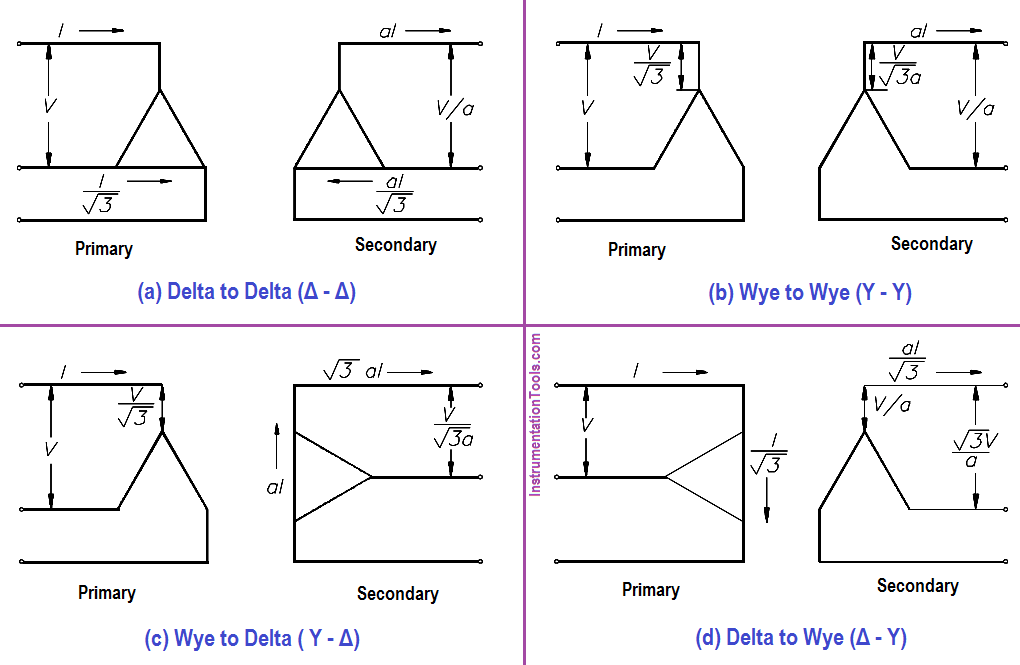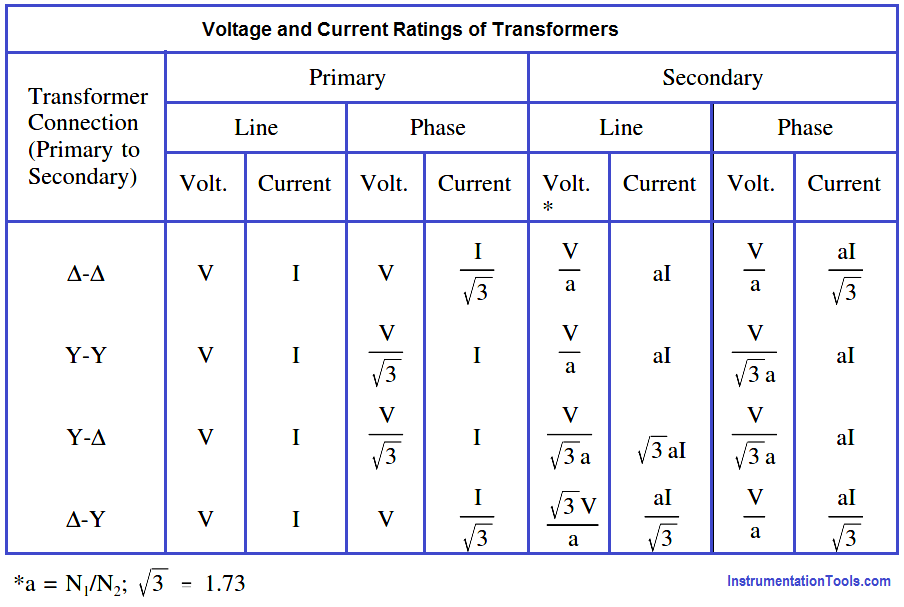# Three Phase Transformer Connections

So far, our discussion has dealt with the operation of single-phase transformers. Three-phase transformer operation is identical except that three single-phase windings are used. These windings may be connected in wye, delta, or any combination of the two.

#### Delta Connection

In the delta connection, all three phases are connected in series to form a closed loop (Figure 1).Figure 1 : Delta Connection

#### Wye Connection

In the wye connection, three common ends of each phase are connected together at a common terminal (marked “N” for neutral), and the other three ends are connected to a three-phase line (Figure 2).Figure 2 :  Wye Connection

#### Combinations of Delta and Wye Transformer Connections

A three-phase transformer may have three separate but identical single-phase (1φ) transformers or a single 3φ unit containing three-phase windings. The transformer windings may be connected to form a 3φ bank in any of four different ways (Figure 3).Figure 3 :  3φ Transformer Connections

Figure 3 shows the voltages and currents in terms of applied line voltage (V) and line current (I), where the turns ratio (a) is equal to one. Voltage and current ratings of the individual transformers depend on the connections (Figure 3) and are indicated by below Table for convenience of calculations.Example 1:

If line voltage is 440 V to a 3φ transformer bank, find the voltage across each primary winding for all four types of transformer connections.

Solution :

Δ – Δ : Primary Voltage = V = 440 Volts

Y – Y : Primary Voltage = V/√3 = 440/1.73 = 254.3 volts

Y – Δ : Primary Voltage = V/√3 = 440/1.73 = 254.3 volts

Δ – Y : Primary Voltage = V = 440 Volts

Example 2:

If line current is 10.4 A in a 3φ transformer connection, find the primary phase current.

Solution :

Δ – Δ : Primary Phase Current = I/√3 = 10.4/1.73 = 6 amps

Y – Y : Primary Phase Current = I = 10.4 amps

Y – Δ : Primary Phase Current = I = 10.4 amps

Δ – Y : Primary Phase Current = I/√3 = 10.4/1.73 = 6 amps

Example 3:

Find the secondary line current and phase current for each type of transformer connection, if primary line current is 20 amps, and the turns ratio is 4:1.

Solution :

∆-∆ : Secondary line current = 4(20) = 80 amps

∆-∆ : Secondary Phase current = aI/√3 = (4×20) / 1.73 = 46.2 amps

Y-Y : Second line current = aI = 4(20) = 80 amps

Y-Y : Second phase current = aI = 4(20) = 80 amps

Y-∆ : Second line current = √3aI = 4(20)(1.73) = 138.4 amps

Y-∆ : Second phase current = aI = 4(20) = 80 amps

∆-Y : Second line current = aI/√3 = (4×20) / 1.73 = 46.2 amps

∆-Y : Second phase current = aI/√3 = (4×20) / 1.73 = 46.2 amps

Don't Miss Our Updates
Be the first to get exclusive content straight to your email.
We promise not to spam you. You can unsubscribe at any time.